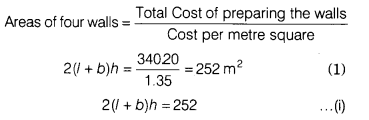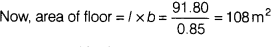# The cost of papering the walls of the room 12 m long at the rate of ₹1.35 per m 2

The cost of papering the walls of the room 12 m long at the rate of ₹1.35 per m 2 is ₹ 340.20 and the cost of matting the floor at the rate of 85 paisa per m 2 is ₹ 91.80 find the height of the room.Also given, the cost of matting the floor at the rate of 85 paisa per m2 is ₹ 91.80.12 X b=108,b=9m
From eqs.(i) and(ii),we get
2(12 x b)h=252